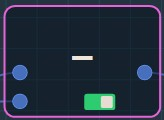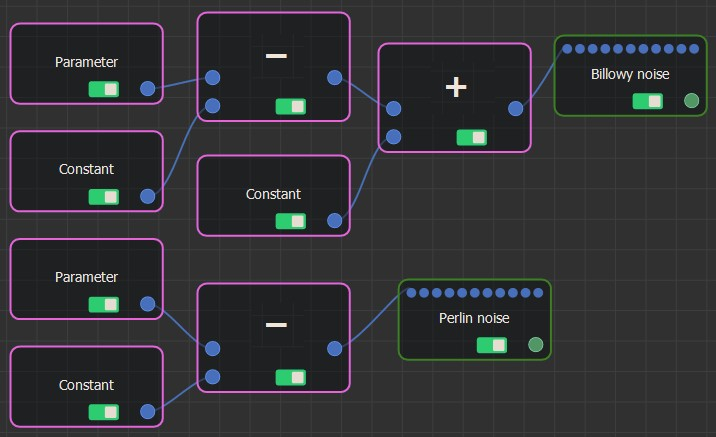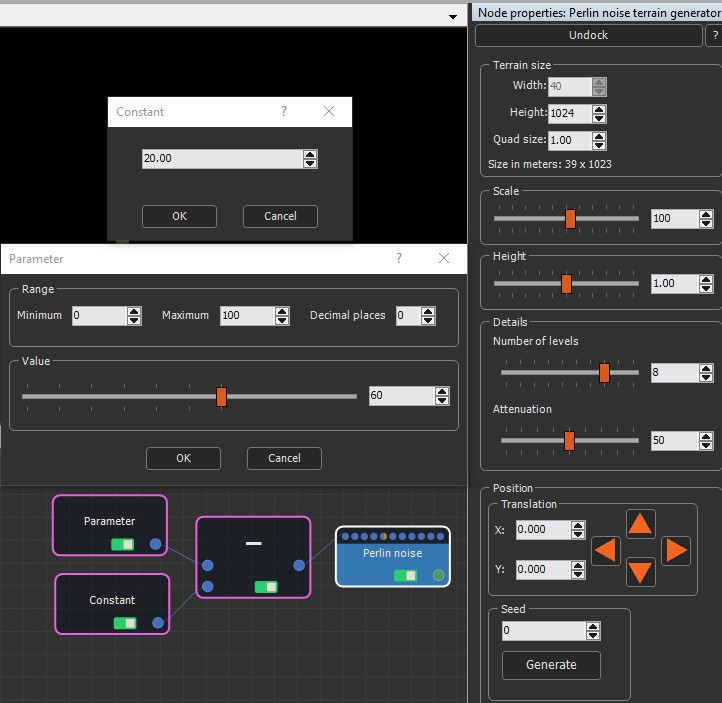### Adding a Minus node

The Minus node subtracts two input numbers to generate an output number. It is an alternative to the Formula node, but allows you to code a formula as a graph rather than a formula.

It has two input connectors and one output connector and subtracts the first input value over the second input value.

To add a Minus node, select Edit in the main menu or right-click in the Graph Editor and select Create Node  > Calculation  > Minus.The Minus node has no parameters.

### Linking a Minus node

The Minus node input connectors link to two input Calculation nodes and the output connector to a Calculation node or an optional connector.### Example

In the example below, the Parameter value and a Constant value are subtracted to drive the terrain size of the Perlin noise. Note that the Terrain size value in the Perlin noise parameters is grayed out because it is replaced by the subtracted values.Copyright © 2021 · All Rights Reserved · Wysilab